# Average diameter of a bounded cell of a simple arrangement

 Importance: Medium ✭✭
 Author(s): Deza, Antoine Terlaky, Tamas Zinchenko, Yuriy
 Subject: Geometry
 Keywords: arrangement diameter polytope
 Posted by: deza on: September 30th, 2007
Conjecture   The average diameter of a bounded cell of a simple arrangement defined byhyperplanes in dimensionis not greater than.

Let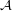be a simple arrangement formed byhyperplanes in dimension. The number of bounded cells ofis. Let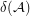denote the average diameter of a bounded cell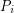of; that is,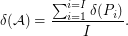Letdenote the largest possible average diameter of a bounded cell of a simple arrangement defined byinequalities in dimension.

We have [DTZ,DX]:

If the conjecture of Hirsch holds, then.for.for.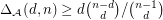for.

## Bibliography

*[DTZ] A. Deza, T. Terlaky and Y. Zinchenko: Polytopes and arrangements : diameter and curvature. Operations Research Letters (to appear).

[DX] A. Deza and F. Xie: Hyperplane arrangements with large average diameter. Centre de Recherches Mathematiques and American Mathematical Society series (to appear).

* indicates original appearance(s) of problem.